Next: Recognition with Invariants Up: Harmonic Ratios and Involutions Previous: The Complete Quadrangle

## Involutions

Definition: An involution is non-trivial projective collineation H whose square is the identity: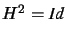.

Property: The mapping taking a point to its harmonic conjugate is an involution.

By the definition of a harmonic pair, the mapping is its own inverse. Using the cross ratio formula (3.2), it is straightforward to check that it is also projective.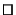Exercise 3.7   :  Consider the affine line, i.e. the projective line with the point at infinity fixed. Show that the only affine involutions (projective involutions fixing the point at infinity) are reflections about a fixed point (e.g., reflection about 0). Deduce that on the projective line, all involutions with one fixed point have two fixed points, and thence that they map points to their harmonic conjugates with respect to these two fixed points.

Exercise 3.8   : (NB: This is an algebraic continuation of the previous exercise). Let a collineation H be represented by a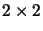matrix as in exercise 3.1. Prove that if H is an involution then a=-d. Show that H always has two distinct fixed points, either both real or both complex. Give an H that fixes the complex points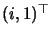and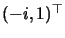, and show that if x'=Hx, then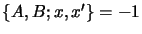. Given the invariance of the cross ratio under complex collineations, show that all involutions of the projective line map points to their harmonic conjugates with respect to the two fixed points of the involution.

Involutions are useful when dealing with images of symmetrical objects. Consider the simple case of a planar object with reflection symmetry, as in fig. 3.5.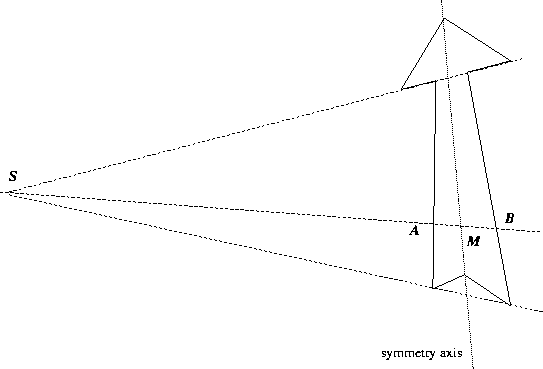In 3D, the lines joining corresponding points of the object are parallel. In the image, all such lines meet at the projection of the associated point at infinity S. The projection of the line of symmetry consists of points conjugate to S with respect of pairs of corresponding points on the shape. There is a plane involution of the image mapping points on the object to their opposites, and fixing Sand each point of the symmetry axis. Also, any line through any two points of the figure meets the line through the two opposite points on the axis: hence the axis is easy to find given a few correspondences. All this extends to 3D symmetries: see  chapter 8.Next: Recognition with Invariants Up: Harmonic Ratios and Involutions Previous: The Complete Quadrangle
Bill Triggs
1998-11-13